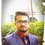# For JEE-2016 (Concepts i forget in Mathematics)

Hello Guys who are giving Jee 2016.

Lets post Some of the things which we used to Forget every time.

Things That I always forget are:

1). Point from which Perpendicular Tangents can be drawn on a curve lies on Director Circle

2). Equation of Hyperbola $\frac { { \quad \left( \quad Distance\quad of\quad point\quad P(x,y)\quad from\quad conjugate\quad Axis \right) }^{ 2 } }{ { \left( \quad Length\quad of\quad semi\quad Transverse\quad axis \right) }^{ 2 } } -\frac { { \left( \quad Distance\quad of\quad point\quad P(x,y)\quad from\quad transverse\quad Axis \right) }^{ 2 } }{ { \left( \quad Length\quad of\quad semi\quad Conjugate\quad axis \right) }^{ 2 } } =1$

3). In hyperbola $|PS-P{ S }^{ ' }|=2b$ Where P is a variable point , $S,{ S }^{ , }$ are the focus and 2b= Length of transverse axis.

4). Standard deviation$\left( \sigma \right)$ or Variance${ \left( \sigma \right) }^{ 2 }$ Do no change on adding or subtracting a number from The observation.

5). Standard Deviation gets multiplied by the number $"h"$ if we multiply the observations by a positive integer $"h"$.

6). $\displaystyle \int { { e }^{ ax }.\sin { \left( bx \right) dx } } =\frac { { e }^{ ax } }{ { a }^{ 2 }+{ b }^{ 2 } } \left( a\sin { \left( bx \right) } -b\cos { \left( bx \right) } \right) \\ \displaystyle \int { { e }^{ ax }.\cos { (bx) } dx } =\frac { { e }^{ ax } }{ { a }^{ 2 }+{ b }^{ 2 } } \left( b\sin { \left( bx \right) } +a\cos { \left( bx \right) } \right)$

7). $\frac { { d }^{ 2 }x }{ d{ y }^{ 2 } } =-\frac { \left( \frac { d{ y }^{ 2 } }{ d{ y }^{ 2 } } \right) }{ { \left( \frac { dy }{ dx } \right) }^{ 3 } }$

8). $\overrightarrow { a } X\left( \overrightarrow { b } X\overrightarrow { c } \right) =\left( \overrightarrow { a } .\overrightarrow { c } \right) \overrightarrow { b } -\left( \overrightarrow { a } .\overrightarrow { b } \right) \overrightarrow { c }$

9). In a triangle ABC $\frac { a }{ \sin { \left( A \right) } } =\frac { b }{ \sin { \left( B \right) } } =\frac { c }{ \sin { \left( C \right) } } =\frac { abc }{ 2\triangle } =2R$

10). In a triangle ABC $\frac { \tan { \left( \frac { B-C }{ 2 } \right) } }{ b-c } =\frac { \tan { \left( \frac { B+C }{ 2 } \right) } }{ b+c } =\frac { \cot { \left( \frac { A }{ 2 } \right) } }{ b+c }$

Please share if you like it.

Also don't Forget to post your concepts that you used to forget at the Papers.Note by Rishabh Deep Singh
5 years, 4 months ago

This discussion board is a place to discuss our Daily Challenges and the math and science related to those challenges. Explanations are more than just a solution — they should explain the steps and thinking strategies that you used to obtain the solution. Comments should further the discussion of math and science.

When posting on Brilliant:

• Use the emojis to react to an explanation, whether you're congratulating a job well done , or just really confused .
• Ask specific questions about the challenge or the steps in somebody's explanation. Well-posed questions can add a lot to the discussion, but posting "I don't understand!" doesn't help anyone.
• Try to contribute something new to the discussion, whether it is an extension, generalization or other idea related to the challenge.

MarkdownAppears as
*italics* or _italics_ italics
**bold** or __bold__ bold
- bulleted- list
• bulleted
• list
1. numbered2. list
1. numbered
2. list
Note: you must add a full line of space before and after lists for them to show up correctly
paragraph 1paragraph 2

paragraph 1

paragraph 2

[example link](https://brilliant.org)example link
> This is a quote
This is a quote
    # I indented these lines
# 4 spaces, and now they show
# up as a code block.

print "hello world"
# I indented these lines
# 4 spaces, and now they show
# up as a code block.

print "hello world"
MathAppears as
Remember to wrap math in $$ ... $$ or $ ... $ to ensure proper formatting.
2 \times 3 $2 \times 3$
2^{34} $2^{34}$
a_{i-1} $a_{i-1}$
\frac{2}{3} $\frac{2}{3}$
\sqrt{2} $\sqrt{2}$
\sum_{i=1}^3 $\sum_{i=1}^3$
\sin \theta $\sin \theta$
\boxed{123} $\boxed{123}$

Sort by:

I do understand 4 and 5, but you could rephrase it for clarity. Can you explain 7 in detail?

- 5 years, 4 months ago

1. Product of the lengths of perpendicular drawn from the focii(both) on any tangent to ellipse (or hyperbola) is $b^2$ b is the length of shorter axis

2. A line that starts from one focus of ellipse after getting reflected from the ellipse , passes through the other focii..

3. For the parabola ,directrix is the director circle.

I have just completed 11th , so, I can help you only with course of 11th, you may ask if you require

Which books are prefered to you in RESONANCE for all the subjects

- 5 years, 4 months ago

They give us Their Own study materials.

- 5 years, 4 months ago

I study in FIITJEE ..we are also given subject materials...but they also tell to refer some subject reference books...I was talking about these books

- 5 years, 4 months ago

S.L. Loney, Calculus in one variable .

But i dont do S.L. loney it is useless .

I like their study material And advanced level problems.

- 5 years, 4 months ago